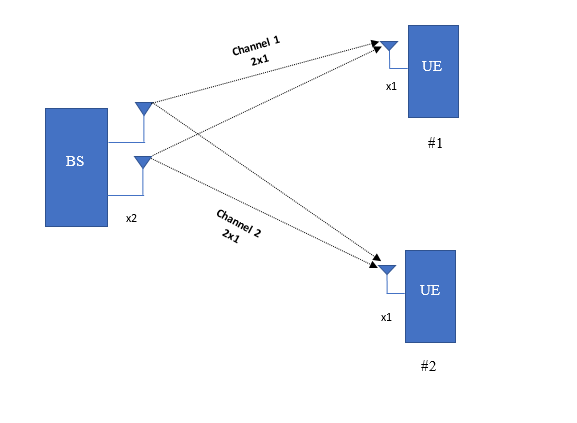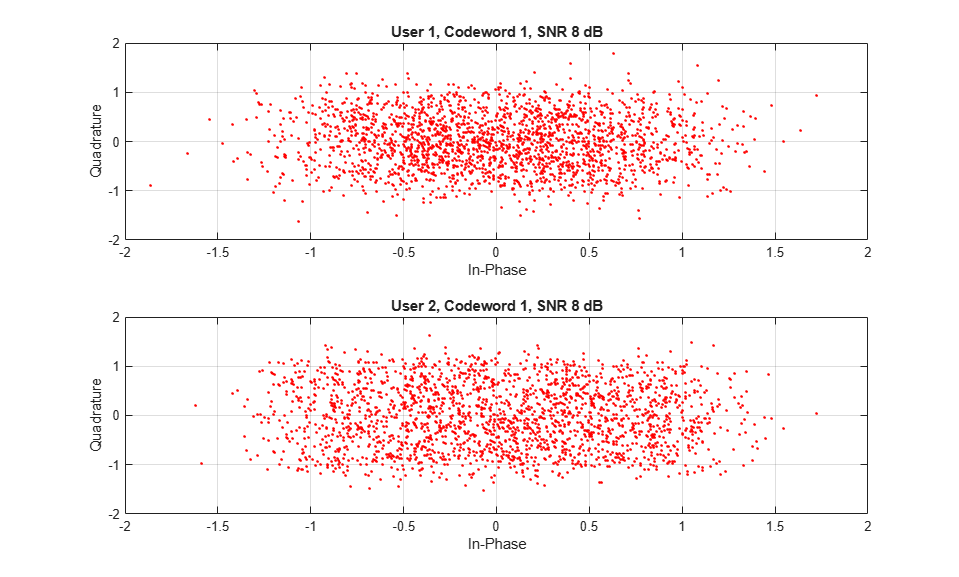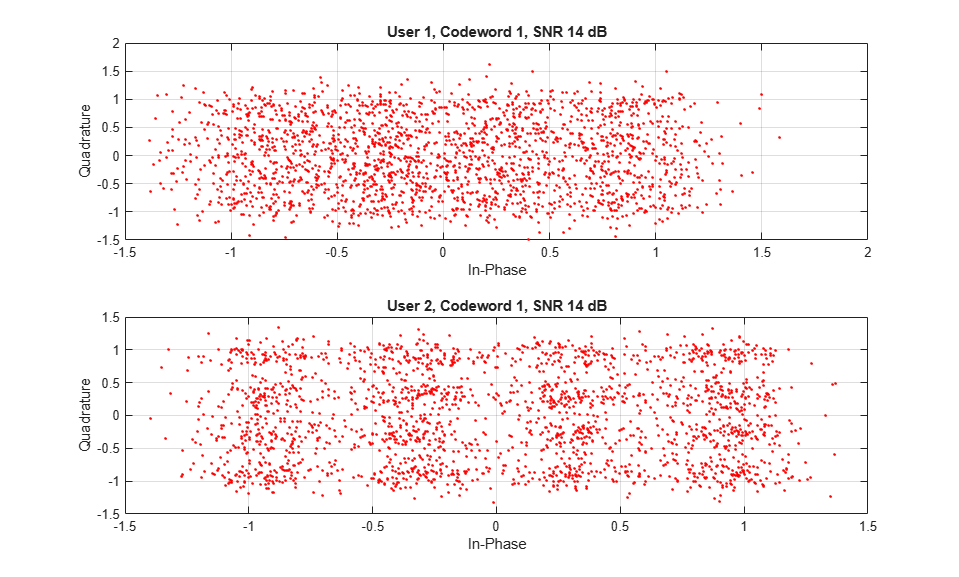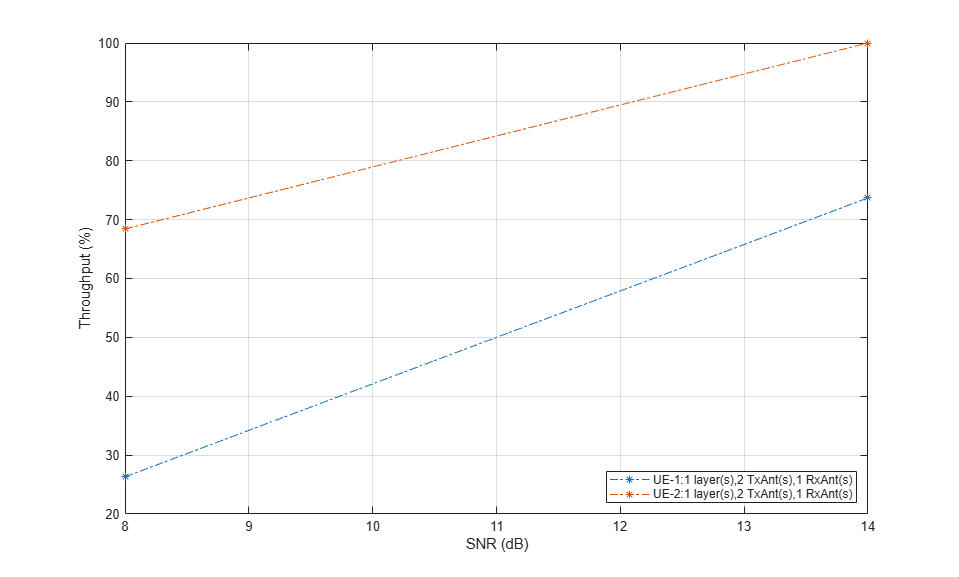Documentation

## PDSCH Throughput for Non-Codebook Based MU-MIMO Transmission Mode 9 (TM9)

This example demonstrates how to measure the physical downlink shared channel (PDSCH) throughput performance in a multiuser multiple-input multiple-output (MU-MIMO) scenario with LTE Toolbox™. It models non-codebook based transmission mode, TM9, with block diagonalization [ 1 ]. This example supports both frequency division duplexing (FDD) and time division duplexing (TDD) schemes. It also supports the use of Parallel Computing Toolbox™ to reduce the effective simulation time.

### Introduction

In a MU-MIMO scenario, due to the simultaneous transmission of data to multiple users, inter-user interference will be present at the receiver. Inter-user interference at the receiver can be canceled using precoding techniques at the transmitter. Two linear precoding techniques for MU-MIMO transmission are channel inversion and block diagonalization. This example uses block diagonalization precoding. This example measures the PDSCH throughput in a MU-MIMO scenario for a number of signal-to-noise ratio (SNR) points. For information on how to model single-user MIMO (SU-MIMO) in LTE look at the following example: PDSCH Throughput for Non-Codebook Based Precoding Schemes: Port 5 (TM7), Port 7 or 8 or Port 7-8 (TM8), Port 7-14 (TM9 and TM10)

A simple MU-MIMO block diagram with the default simulation configuration parameters used in the example is shown in the following figure.### Simulation Configuration

The simulation parameters for the base station and users are configured in this section. The example is executed for a simulation length of two frames for a number of SNR points. Increase `NFrames` to increase the simulation time and produce statistically significant throughput results. Use the variable `SNRIn` to set the SNR, it can be an array of values or a scalar. As per the constraints in LTE [ 2 ], this example supports a maximum of 4 users with a maximum of 4 layers across all users. The number of maximum layers per user is 2. The number of transmit antennas should be greater than or equal to the total number of receive antennas across all users.

```NFrames = 2; % Number of frames SNRIn = [8 14]; % SNR range in dB NUsers = 2; % Number of active users NTxAnts = 2; % Number of antennas at eNodeB % Specify UE-specific parameters muNumLayers = [1 1 1 1]; % Number of layers for a maximum of 4 users muNumRxAnts = [1 1 1 1]; % Number of receive antennas for a maximum of 4 users muCodeRate = [0.5 0.5 0.5 0.5]; % Code rate for a maximum of 4 users muModulation = {'16QAM';'16QAM';'16QAM';'16QAM'}; % Modulation for a maximum of 4 users ```

The set of parameters required for TM9 is specified below. This example does not perform DCI format decoding; the `DCIFormat` field is included for completeness. The cell array `muPDSCH` stores the PDSCH transmission configuration structure for all users.

```% Initialize cell arrays of PDSCH transmission configuration structures, % transport block sizes and coded transport block sizes. muPDSCH = cell(NUsers,1); trBlkSizes = cell(NUsers,1); codedTrBlkSizes = cell(NUsers,1); simulationParameters = []; % clear simulation parameters simulationParameters.NDLRB = 50; simulationParameters.PDSCH.PRBSet = (0)'; simulationParameters.PDSCH.DCIFormat = 'Format2C'; simulationParameters.PDSCH.TxScheme = 'Port7-14'; simulationParameters.PDSCH.NTxAnts = NTxAnts; simulationParameters.DuplexMode = 'FDD'; % 'FDD', 'TDD' simulationParameters.TotSubframes = 1; % PDSCH configuration structure for users based on the common and % user-specific parameters ncw = zeros(NUsers,1); for userIdx = 1:NUsers simulationParameters.PDSCH.TargetCodeRate = muCodeRate(userIdx); simulationParameters.PDSCH.Modulation = muModulation{userIdx}; simulationParameters.PDSCH.NLayers = muNumLayers(userIdx); % Initialize W to zero simulationParameters.PDSCH.W = zeros(muNumLayers(userIdx),NTxAnts); % Downlink reference measurement channel configuration enb = lteRMCDL(simulationParameters); % PDSCH transmission configuration structure for users muPDSCH{userIdx}= enb.PDSCH; % Number of codewords for users ncw(userIdx) = length(muPDSCH{userIdx}.Modulation); % Store transport block sizes for users trBlkSizes{userIdx} = muPDSCH{userIdx}.TrBlkSizes; end % Assign redundancy version sequence rvSequence = muPDSCH{1}.RVSeq; ```

Print a summary of some of the more relevant simulation parameters.

```hMultiUserParameterSummary(enb,muPDSCH,muNumRxAnts); ```
``` Parameter summary for TM9 MU-MIMO Transmission ------------------------------------------------------------------ Duplexing mode: FDD Transmission mode: TM9(MU-MIMO) Transmission scheme: Port7-14 Number of downlink resource blocks: 50 Number of allocated resource blocks: 1 Number of transmit antennas: 2 ------------------------------------------------------------------ Number of Transmission layers for UE-1: 1 Number of codewords for UE-1: 1 Number of receive antennas for UE-1: 1 Modulation codeword 1: 16QAM Transport block sizes codeword 1: 208 208 208 208 208 208 208 208 208 208 Code rate codeword 1: 0.5088 0.5088 0.5088 0.5088 0.5088 0.5088 0.5088 0.5088 0.5088 0.5088 ------------------------------------------------------------------ Number of Transmission layers for UE-2: 1 Number of codewords for UE-2: 1 Number of receive antennas for UE-2: 1 Modulation codeword 1: 16QAM Transport block sizes codeword 1: 208 208 208 208 208 208 208 208 208 208 Code rate codeword 1: 0.5088 0.5088 0.5088 0.5088 0.5088 0.5088 0.5088 0.5088 0.5088 0.5088 ```

### Propagation Channel

The channel model configuration parameters for the channel between the eNodeB and the users are stored in the cell array `muChannel`. The set of common parameters for each channel is initially specified. The parameters defined here are used with `lteFadingChannel` during subframe processing.

```muChannel = cell(NUsers,1); channel = struct; channel.DelayProfile ='EPA'; % Delay profile channel.MIMOCorrelation = 'Low'; % Multi-antenna correlation channel.NTerms = 16; % Oscillators used in fading model channel.ModelType = 'GMEDS'; % Rayleigh fading model type channel.InitPhase = 'Random'; % Random initial phases channel.NormalizePathGains = 'On'; % Normalize delay profile power channel.NormalizeTxAnts = 'On'; % Normalize for transmit antennas ```

The channel sampling rate depends on the FFT size used in the OFDM modulator. This can be obtained using the function `lteOFDMInfo`.

```ofdmInfo = lteOFDMInfo(enb); channel.SamplingRate = ofdmInfo.SamplingRate; % Independent channel configuration parameters for each user chanSeeds = [1111 2222 3333 4444]; % Channel seed for a maximum of 4 users dopplerFreq = [5 50 25 15]; % Doppler frequency for a maximum of 4 users for userIdx = 1:NUsers muChannel{userIdx}= channel; muChannel{userIdx}.Seed = chanSeeds(userIdx); % Channel seed muChannel{userIdx}.NRxAnts = muNumRxAnts(userIdx); % Number of receive antennas muChannel{userIdx}.DopplerFreq = dopplerFreq(userIdx);% Doppler frequency end ```

### Processing Chain

To determine the throughput at each SNR point, the subframe-by-subframe PDSCH processing chain includes:

• Calculating the Precoding Matrix - A perfect channel estimate is used to calculate the precoding matrix for each user. A detailed explanation of this step is provided in the next section.

• Updating Current HARQ Process - Separate HARQ processes are used for each user.

• Creating Transmit Waveform - Separate PDSCH symbols are generated for each user. The PDSCH symbols are precoded with the calculated precoding matrix. The precoded PDSCH symbols corresponding to the UEs are combined and OFDM modulated.

• Channel Modeling - Pass the waveform through a fading channel to each user and add noise (AWGN)

• Performing Synchronization and OFDM Demodulation - Separately carried out for each user. Offset the received symbols to account for a combination of implementation delay and channel delay spread. OFDM demodulate the symbols.

• Decoding the PDSCH - Separately carried out for each user. Perfect channel estimate is assumed at the receiver for decoding operations. Obtain an estimate of the received codewords using `ltePDSCHDecode` to demodulate and descramble the recovered PDSCH symbols for all transmit and receive antenna pairs.

• Decoding the DL-SCH and Storing the Block CRC - Separately carried out for each user. Pass the vector of decoded soft bits to `lteDLSCHDecode`, which decodes the codeword and returns the block CRC error used to determine the throughput of the system.

### Precoding Matrix Calculation

The precoding matrix for each user needs to be computed based on the channel estimates between the eNodeB and users. The precoding matrix is calculated using the block diagonalization method. For the computation of the precoding matrix, channel state information (CSI) is required at the transmitter. In this example, for the sake of simplicity, perfect knowledge of the channel between the eNodeB and the users is assumed at the base station.

For TDD, the channel estimates between the eNodeB and the users are estimated in the last UL subframe before a DL subframe. These channel estimates are used to calculate the precoding matrix, `W`. All subsequent DL subframes (including special subframes) until the next UL subframe are precoded with matrix `W`.

For FDD, there is a delay of one subframe between the calculation of `W` and the subframe where it is used. For example, `W` used in DL subframe `n` has been calculated with the channel estimates obtained in DL subframe `n-1`.

The function hMultiUserPrecodingMatrix calculates `W` as shown in the figure below:

• Averaging the perfect channel estimates for all the allocated RBs

• Calculating the augmented channel matrix (Htilde, matrix with channel gains of all users except for the user of interest)

• Computing the right null space (V1) of the augmented channel matrix using singular value decomposition

• Multiplying the channel matrix of the user of interest (Hu) and the right null space of the augmented channel matrix to obtain the resulting channel matrix (Hu*V1)

• Calculating the singular value decomposition of the resulting channel matrix (Hu*V1) and extracting the first `NLayers` columns (V2)

• Multiplying V1 and V2 to obtain `W` for the user of interestNote that for an allocation of a single resource block, the precoding matrix will usually be well matched to the channel conditions, with little deviation from the optimal precoding. But as the allocation size increases, the precoding matrix takes into account the average of the channel conditions over the whole allocation. This averaging causes a deviation from the optimal precoding matrix. Therefore, you can expect a degradation in performance as the size of the resource allocation increases.

### Processing Loop

The 'for' loop for SNR points processing is included below. To enable the use of parallel computing for increased speed use 'parfor' instead of 'for' in the loop. This requires the Parallel Computing Toolbox. If this is not installed 'parfor' will default to the normal 'for' statement.

```% Initialize variables used in the simulation and analysis maxThroughput = zeros(length(SNRIn),NUsers); simThroughput = zeros(length(SNRIn),NUsers); harqProcesses = cell(NUsers,1); % Initialize cell array for constellation plot rxConstellation = cell(numel(SNRIn),NUsers,2); % Copy the channel cell array and cell array of PDSCH transmission % configuration structure to optimize parallel processing (only if running % the example with Parallel Computing Toolbox) muChannelInit = muChannel; muPDSCHInit = muPDSCH; % During the simulation, some fields of enb will be updated, make a copy to % reinitialize it when simulating each SNR point enbInit = enb; % For TDD precalculate vector of subframe types: D, S and U for downlink, % special, and uplink, respectively if strcmpi(enb.DuplexMode,'TDD') subframeType = char(10,1); initialSubframeNo = enb.NSubframe; for sNo=0:9 % for all subframes in a frame enb.NSubframe = sNo; duplexInfo = lteDuplexingInfo(enb); subframeType(sNo+1) = duplexInfo.SubframeType(1); % first char: D, S or U end enb.NSubframe = initialSubframeNo; end % CFI can be a scalar, or a vector of length 10 (corresponding to a frame) % if the CFI varies per subframe. If CFI is scalar, create a local copy of % CFI as a vector (one value per subframe). if numel(enb.CFI) == 1 CFI = repmat(enb.CFI,1,10); else CFI = enb.CFI; end for snrIdx = 1:numel(SNRIn) % Comment out for parallel computing %parfor snrIdx = 1:numel(SNRIn) % Uncomment for parallel computing % Set the random number generator seed depending on the loop variable % to ensure independent random streams rng(snrIdx,'combRecursive'); % Reinitialize enb structures (they are modified during % each SNR point simulation) enb = enbInit; % Reinitialize muChannel and muPDSCH cell array muChannel = muChannelInit; muPDSCH = muPDSCHInit; % Initialize the state of all HARQ processes harqProcesses = cell(NUsers,1); for userIdx = 1:NUsers harqProcesses{userIdx} = hNewHARQProcess(enb,muPDSCH{userIdx}); end harqProcessSequence = 1; % Set up variables for the main loop lastOffset = zeros(NUsers,1); frameOffset = zeros(NUsers,1); blkCRC = []; rxSymbols = cell(NUsers,2); % DL-SCH symbols for constellation plot bitTput = cell(NUsers,1); txedTrBlkSizes = cell(NUsers,1); W = cell(NUsers,1); pdschIndices = []; pdschRho = 0; % Flag to indicate if a precoding matrix cell array W is available. isWready = false; % Flag to indicate if a subframe is to be processed. Set to true if % there is data to be processed in the subframe, i.e. non-zero transport % block size. processSubframe = false; % Main for loop: for all subframes for subframeNo = 0:(NFrames*10-1) % Update subframe number enb.NSubframe = subframeNo; % Load CFI for current subframe enb.CFI = CFI(mod(subframeNo,length(CFI))+1); % Get HARQ process ID for the subframe from HARQ process sequence harqID = harqProcessSequence(mod(subframeNo,... length(harqProcessSequence))+1); % Channel fading process time offset for the current subframe and % transport block size(s) trBlk = zeros(NUsers,2); % User can have maximum 2 transport block trBlkNext = zeros(NUsers,2); for userIdx = 1:NUsers % Initialize channel fading process time offset for each subframe muChannel{userIdx}.InitTime = subframeNo/1000; trBlk(userIdx,1:ncw(userIdx)) = trBlkSizes{userIdx}(:,mod(subframeNo, 10)+1).'; % Get transport block for next subframe trBlkNext(userIdx,1:ncw(userIdx))= trBlkSizes{userIdx}(:,mod(subframeNo+1,10)+1).'; end % Set the flag to trigger subframe processing if isWready && any(trBlk(:)) processSubframe = true; else processSubframe = false; end % Precoding matrix calculation if strcmpi(enb.DuplexMode,'TDD') % Estimate channel in UL subframe if strcmp(subframeType(mod(subframeNo,10)+1),'U') processSubframe = false; % UL subframe, no DL data % Only perform channel estimate if next subframe is DL if strcmp(subframeType(mod((subframeNo+1),10)+1),'D') W = hMultiUserPrecodingMatrix(enb,muPDSCH,muChannel); isWready = true; end end else %FDD % Calculate the precoding matrix for next subframe only if it % carries data (i.e. non-zero trBlkNext) if any(trBlkNext(:)) W = hMultiUserPrecodingMatrix(enb,muPDSCH,muChannel); isWready = true; else isWready = false; end end % Subframe processing if processSubframe % In this example, the variables pdschRho and pdschIndices will % have the same values for all users codedTrBlk = zeros(NUsers,2); for userIdx = 1:NUsers % Update current HARQ process for all users harqProcesses{userIdx}(harqID) = hHARQScheduling( ... harqProcesses{userIdx}(harqID), subframeNo, rvSequence); % Map precoding matrix of all users into PDSCH configuration % cell array muPDSCH{userIdx}.W = W{userIdx}; % PDSCH resource element power allocation in dB pdschRho = muPDSCH{userIdx}.Rho; % Generate indices for mapping of PDSCH symbols on resource % grid [pdschIndices,pdschInfo] = ltePDSCHIndices(enb,... muPDSCH{userIdx},muPDSCH{userIdx}.PRBSet); % Obtain coded transport block size codedTrBlk(userIdx,1:ncw(userIdx)) = pdschInfo.G; end % Generate grid without any PDSCH mapped [~,txGrid,enbOut] = lteRMCDLTool(enb,[]); % Get the HARQ ID sequence from 'enbOut' for HARQ processing harqProcessSequence = enbOut.PDSCH.HARQProcessSequence; % Generate complex-valued modulated symbol for PDSCH in multi-user % MIMO transmission with block-diagonalization precoding pdschSymbols = hMultiUserPDSCH(enb,muPDSCH,codedTrBlk,... harqProcesses,harqID); powerAdjPerRE = 10^(pdschRho/20); % Perform PDSCH symbols mapping on resource grid txGrid(pdschIndices) = pdschSymbols*powerAdjPerRE; % Perform OFDM modulation [waveform,ofdmInfo] = lteOFDMModulate(enb,txGrid); % Add 25 sample padding. This is to cover the range of delays % expected from channel modeling (a combination of % implementation delay and channel delay spread) txWaveform = [waveform; zeros(25,NTxAnts)]; % Calculate noise gain including compensation for downlink % power allocation SNR = 10^((SNRIn(snrIdx)-muPDSCH{userIdx}.Rho)/20); % Normalize noise power to take account of sampling rate, % which is a function of the IFFT size used in OFDM % modulation, and the number of antennas N0 = 1/(sqrt(2.0*NTxAnts*double(ofdmInfo.Nfft))*SNR); % Pass the waveform through noisy fading channels and receiver % operations for each user for userIdx = 1:NUsers % Pass data through channel model rxWaveform = lteFadingChannel(muChannel{userIdx},txWaveform); % Create additive white Gaussian noise noise = N0*complex(randn(size(rxWaveform)), ... randn(size(rxWaveform))); % Add AWGN to the received time domain waveform rxWaveform = rxWaveform + noise; % Receiver % Synchronization offset, OFDM demodulation, % perfect channel estimation, PDSCH and DL-SCH Decoding [harqProcesses{userIdx},dlschSymbols,lastOffset(userIdx)]... = hReceiverOperations(enb,muPDSCH{userIdx},rxWaveform,... muChannel{userIdx},harqProcesses{userIdx},trBlk(userIdx,... 1:ncw(userIdx)),lastOffset(userIdx),harqID,subframeNo,noise); % Store the decoded DLSCH symbols for constellation % plotting rxSymbols{userIdx,1} = [rxSymbols{userIdx,1}; dlschSymbols{1}(:)]; if ncw(userIdx)>1 rxSymbols{userIdx,2} = [rxSymbols{userIdx,2}; dlschSymbols{2}(:)]; end % Store values to calculate throughput % Only for subframes with data if(any(trBlk(userIdx,1:ncw(userIdx))) ~= 0) blkCRC = [blkCRC harqProcesses{userIdx}(harqID).blkerr]; bitTput{userIdx} = [bitTput{userIdx} ... trBlk(userIdx,1:ncw(userIdx)).*(1-harqProcesses{userIdx}(harqID).blkerr)]; txedTrBlkSizes{userIdx} = [txedTrBlkSizes{userIdx} ... trBlk(userIdx,1:ncw(userIdx))]; end end end end % Calculate the maximum and simulated throughput maxTput = zeros(NUsers,1); simTput = zeros(NUsers,1); for userIdx = 1:NUsers maxTput(userIdx) = sum(txedTrBlkSizes{userIdx}); % Max possible throughput simTput(userIdx) = sum(bitTput{userIdx},2); % Simulated throughput % Display the results dynamically in the command window fprintf('\nSNR = %.2f dB. Throughput for UE-%d %d Frame(s) = %.4f Mbps\n',... SNRIn(snrIdx),userIdx,NFrames,1e-6*simTput(userIdx)/(NFrames*10e-3)); fprintf('SNR = %.2f dB. Throughput(%%) for UE-%d %d Frame(s) = %.4f %%\n',... SNRIn(snrIdx),userIdx, NFrames,simTput(userIdx)*100/maxTput(userIdx)); end maxThroughput(snrIdx,:) = maxTput; simThroughput(snrIdx,:) = simTput; rxConstellation(snrIdx,:,:)= rxSymbols; end % Plot received symbol constellation for snrIdx = 1:numel(SNRIn) ii = 1; figure; for userIdx = 1:NUsers subplot(NUsers,max(ncw),ii); plot(rxConstellation{snrIdx,userIdx,1},'.r'); title(['User ' num2str(userIdx) ', Codeword 1, SNR '... num2str(SNRIn(snrIdx)) ' dB']); xlabel('In-Phase'); ylabel('Quadrature'); grid on; if size(rxConstellation{snrIdx,userIdx,2})~=0 ii = ii+1; subplot(NUsers,max(ncw),ii); plot(rxConstellation{snrIdx,userIdx,2},'.r'); title(['User ' num2str(userIdx) ', Codeword 2, SNR '... num2str(SNRIn(snrIdx)) ' dB']); xlabel('In-Phase'); ylabel('Quadrature'); grid on; end ii = ii+1; end end ```
```SNR = 8.00 dB. Throughput for UE-1 2 Frame(s) = 0.0520 Mbps SNR = 8.00 dB. Throughput(%) for UE-1 2 Frame(s) = 26.3158 % SNR = 8.00 dB. Throughput for UE-2 2 Frame(s) = 0.1352 Mbps SNR = 8.00 dB. Throughput(%) for UE-2 2 Frame(s) = 68.4211 % SNR = 14.00 dB. Throughput for UE-1 2 Frame(s) = 0.1456 Mbps SNR = 14.00 dB. Throughput(%) for UE-1 2 Frame(s) = 73.6842 % SNR = 14.00 dB. Throughput for UE-2 2 Frame(s) = 0.1976 Mbps SNR = 14.00 dB. Throughput(%) for UE-2 2 Frame(s) = 100.0000 % ```### Throughput Results

The throughput results for all users are displayed in the MATLAB® command window after the simulation for each SNR point is completed. They are also captured in output arrays `simThroughput` and `maxThroughput`.

```legendString = cell(NUsers,1); figure; for userIdx = 1:NUsers plot(SNRIn, simThroughput(:,userIdx)*100./maxThroughput(:,userIdx),'*-.'); hold on; legendString{userIdx} = strcat('UE-' ,num2str(userIdx), ': ', ... num2str(muNumLayers(userIdx)), ' layer(s), ' ,num2str(NTxAnts), ... ' TxAnt(s), ', num2str(muNumRxAnts(userIdx)), ' RxAnt(s)'); end grid on; xlabel('SNR (dB)'); ylabel('Throughput (%)'); legend(legendString,'Location','SouthEast'); ```For statistically valid results, the simulation should be run for a larger number of frames. The figure below shows the throughput results when simulating 1000 frames.### Appendix

This example uses the following helper functions:

### Selected Bibliography

1. Spencer Q., A. Swindlehurst, M. Haardt. "Zero-Forcing Methods for Downlink Spatial Multiplexing in Multiuser MIMO Channels." IEEE Transactions on Signal Processing, Vol. 52, No. 2, February 2004, pp. 461-471.

2. Lim C., T. Yoo, B. Clerckx, B. Lee, B. Shim. "Recent trend of multi-user MIMO in LTE-advanced." IEEE Communications Magazine, March 2013, pp. 127-135.